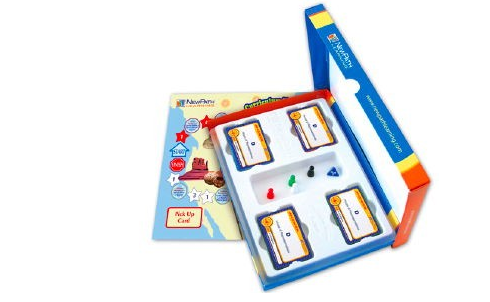# Tất cả sản phẩm# Grade 6 Math Curriculum Mastery® Game - Study-Group Edition

Mã sản phẩm: 23-6401 Lược xem: 1131

Liên hệ

Includes one double-sided game board, a blended set of 250 cards representing a cross-section of the topics represented in the Class-Pack Edition (23-6001), materials for four students, and trial online subscription. Topics include:

1. Whole Numbers to Trillions
2. Commutative/Associative Properties
3. Distributive Property
4. Equivalent Fractions
5. Percents
6. Ordering Rational Numbers
8. Multiply/Divide Fractions
9. Mixed Numbers
10. Multiple Representation of Rational Numbers
11. Percent, Rate, Base
12. Order of Operations
13. Repeated Multiplication to Exponents
14. Exponents to Repeated Multiplication
15. Evaluate Exponents
16. One & Two Step Equations
17. Evaluate Formulas
18. Simple Proportions
19. Area of Triangles & Quadrilaterals
20. Area & Circumference of Circles
21. Plotting Points
22. Area of Coordinate Polygons
23. Measurement Conversion
24. Statistics & Probability
25. Graphs